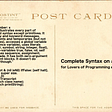# C++ PROGRAM TO FIND THE ARMSTRONG NUMBER!!!

|ARMSTRONG NUMBER|

In this program, we are going to find the ARMSTRONG NUMBER. Let’s first understand what’s Armstrong number🤔

ARMSTRONG NUMBER:

Is the number that is equal to the sum of cubes of its digits. For example 0, 1, 153, 370, 371, and 407 are the Armstrong numbers.

*Let’s try to understand why 153 is an Armstrong number.

153=(1*1*1)(5*5*5)(3*3*3)

(1*1*1)=1

(5*5*5)=125

(3*3*3)=27

• Let’s see the c++ program to check Armstrong's number.

“#include<iostream>” →Input-output continuous bytes of data.

“using namespace std;” →used to store variable name.

“int main()” →returns datatype function.

“{ int n,r,sum=0,temp;
cout<<”Enter the Number= “;
cin>>n;
temp=n;”

• First, we are defining four variables in integer (n,r,sum=0, temp) because we are going to get a number; In cout statement, we have given “Enter the Number=”; then we were given temp=n

For example: if we give n=13 so it takes the same value in another variable(i.e.,”temp=13”)

“while(n>0)
{
r=n%10;
sum=sum+(r*r*r);
n=n/10;
}”

WHILE LOOP:

The syntax of a while loop in C++ is − while(condition) { statement(s); } Here, statement(s) may be a single statement or a block of statements. The condition may be any expression, and true is any non-zero value. The loop iterates while the condition is true.

• In the while loop we gave n is greater than 0; if the given number is greater than 0 it will enter the while loop.

“if(temp==sum)
cout<<”Armstrong Number.”<<endl;
else
cout<<”Not Armstrong Number.”<<endl;
return 0;
}”

IF STATEMENT:

C++ has the following conditional statements: Use if to specify a block of code to be executed, if a specified condition is true. Use else to specify a block of code to be executed, if the same condition is false. Use else if to specify a new condition to test, if the first condition is false.

• If the value of temp and sum is equal it will print “Armstrong number”; Otherwise it will print “not Armstrong number”

endl →avoid buffer statement and display in new line.

return 0 →that means the program is executed successfully.

## OUTPUT:

IF THE NUMBER IS ARMSTRONG.

IF THE NUMBER IS NOT ARMSTRONG.

--

--

--

## More from Gomathi Keerthana.R.S.

Love podcasts or audiobooks? Learn on the go with our new app.

## Configuring prometheus-operator helm chart with AWS EKS## Azure Event Grid — Handling Failures## Algorithm Question: Climbing Stairs## Week 2 Reflection #GenerasiGIGIH Front End Engineering Path## The Cloud — Part #1 | Intro + Mental Model## SUGAR BOUNCE AMA RECAP## LGM-VIP July 2021## Building Data products in 2018## What is c Programming?## When Pigeons Talk: The Evolution of Programming Education## All about Static Libraries in C## What You Need To Get About Git Before Gitting…GETTING Started